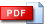# MATH241 - Ordinary Differential Equations and Linear Algebra

### The Aim of the Course

There are two objectives of this course: the first, to understand the basic concepts and skills of linear algebra which are required for simple applications in science and engineering problems, and the second, to learn some elementary methods for solving first and second-order differential equations, and apply linear algebra to solve first-order systems of linear differential equations.
The Topics are; Systems of linear equations, elementary row operations, echelon form, Gaussian elimination method; Matrices; Determinants, adjoint and inverse matrices, Cramer’s rule; Vector spaces, linear independence, bases and dimension, eigenvalue problem.

First-order differential equations, separable differential equations, change of variables, exact differential equations; Second-order differential equations, the method of undetermined coefficients, the variation of parameters method; General results of first-order linear systems, homogeneous constant coefficient vector differential equations, variations of parameters for linear systems; Laplace transform method.

### Instructors

Dr. F. B. Rizaner (01), Dr. xx

### Lecture Notes

 Lecture 1Lecture 2